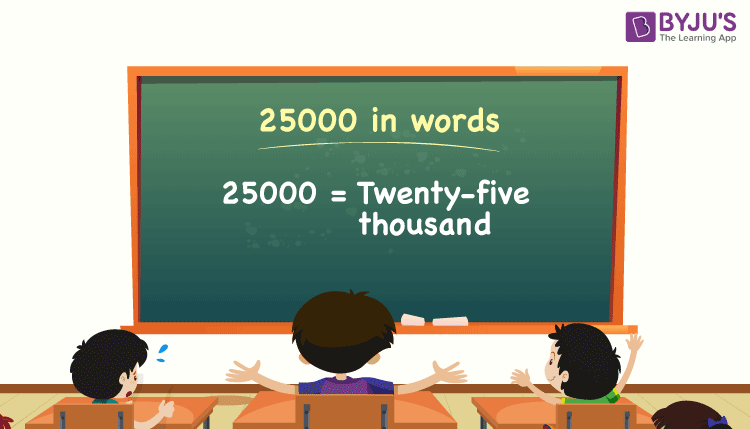# 25000 in Words

25000 in words can be written as Twenty-five Thousand. Suppose we bought a refrigerator worth Rs. 25,000, we can communicate this to friends as “We bought a refrigerator that costs Twenty-five thousand”. Also, we know that 25000 is a cardinal number since it represents a specific quantity.

 25000 in words: Twenty-five thousand Twenty-five thousand in numerical form: 25000

25000 in English words

We generally write numbers in words using the English alphabet. Thus, we can read 25000 in English as “Twenty-five Thousand”.## How to Write 25000 in Words?

We can convert 25000 to words using a place value chart. Let’s have a look at the conversion of the number 25000 to words.

The number 25000 has 5 digits so let’s make a chart which shows the place value up to 5 digits.

 Ten thousand Thousands Hundreds Tens Ones 2 5 0 0 0

Thus, we can write the expanded form as:

2 × Ten thousand + 5 × Thousand + 0 × Hundred + 0 × Ten + 0 × One

= 2 × 10000 + 5 × 1000 + 0 × 100 + 0 × 10 + 0 × 1

= 20000 + 5000

= 25000

= Twenty-five thousand

Hence, in words, 25000 is written as ”Twenty-five thousand”.

Interesting way of writing 25000 in words:

2 = Two

25 = Twenty-five

250 = Two hundred and fifty

2500 = Two thousand five hundred

25000 = Twenty-five thousand

25000 is the natural number that is succeeded by 24999 and preceded by 25001.

25000 in words – Twenty-five thousand

Is 25000 an odd number? – No

Is 25000 an even number? – Yes

Is 25000 a perfect square number? – No

Is 25000 a perfect cube number? – No

Is 25000 a prime number? – No

Is 25000 a composite number? – Yes

## Frequently Asked Questions on 25000 in Words

Q1

### What is the correct spelling of 25000?

The spelling of 25000 in English words is Twenty-five thousand, which means we can express 25000 in words as twenty-five thousand.

Q2

### How do you write 25000 rupees in words?

25000 rupees in words can be written as Twenty-five thousand rupees.

Q3

### How do you write 25000 in words on a cheque?

On a cheque, we generally write 25000 in words as Twenty-five thousand rupees only.

Q4

### How do you say 25000?

We can say 25000 in words as twenty-five thousand. If this number is used to represent the money or the cost of something, then we pronounce it as twenty-five thousand rupees.

Test your Knowledge on 25000 in Words